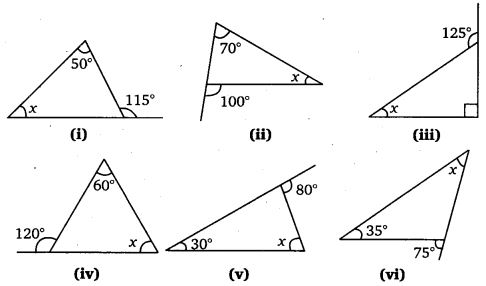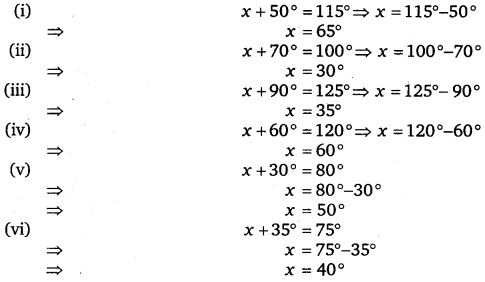# Class 7 Maths NCERT Solutions for Chapter – 6 The Triangle and its Properties Ex – 6.2

### The Triangle and its Properties

Question 1.
Find the value of the unknown exterior angle x in the following diagrams :Solution:
Since, in a triangle an exterior angle is equal to the sum of the two interior opposite angles, therefore,

1. x = 50°+ 70° = 120°
2. x = 65°+ 45° = 110°
3. x = 30°+ 40°= 70°
4. x = 60° + 60° = 120c
5. x = 50° + 50° = 100c
6. x = 30°+ 60° = 90°

Question 2.
Find the value of the unknown interior angle x in the following figures :Solution:
We know that in a triangle, an exterior angle is equal to the sum of the two interior opposite angles. Therefore,